Example : Seepage Pressure And Quicksand

Problem : In a geotechnical survey it was found that a soil layer, that is 6 m thick and has the porosity of 45%, lies on top of an aquifer. The upward seepage head at the bottom of the soil layer was found to be 5m.

Determine the critical hydraulic gradient of soil.

Soil has to be excavated to lay the foundation of a structure. Find out the maximum depth up to which the excavation can be safely carried out. What should be the depth of excavation so that the danger of the bottom becoming unstable under the artesian pressure in the sand can be avoided.

Consider specific gravity of the particles of the soil is 2.7 and unit weight of water 9.81 KN/m3.

Solution:

let's illustrate the problem: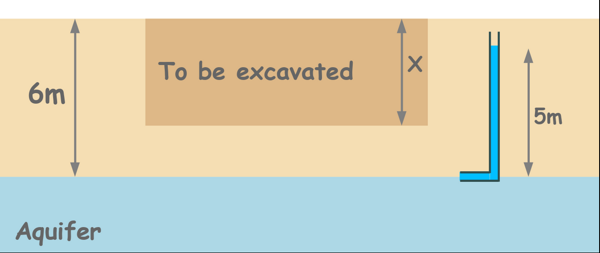Given in problem :

porosity of soil mass : n = 45%

Specific Gravity of Solids : Gs = 2.7

Unit weight of Water : γw = 9.81 KN/m3

Upward seepage head at the bottom of the soil layer : h = 5m.

First we will calculate the critical hydraulic gradient for the soil mass.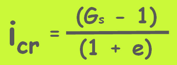............................(1)

we have the Gs but first we need to calculate the void ratio e.

we know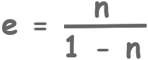............................(2)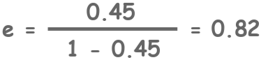now substitute this in the equation number 1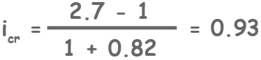To calculate the safe excavation depth let's imagine we have already excavated the soil up to the depth X. Now the remaining soil should be able to exert enough pressure downward to withstand the upward artesian pressure. If we excavate further, upward pressure will become dominant and the surface will become unstable.

Pressure exerted by the remaining soil at the bottom of the soil will be unit weight of soil γ multiplied by the height of the remaining soil (6 - X).

And upward pressure because of upward seepage head is unit weight of water γw multiplied by the head 5m.

γ(6 - X) = γw5   ............................(3)

For this calculation we have all the parameters but the unit weight of soil.

For that let's assume the soil mass is saturated because water is seeping upwards and some water must have percolated through its voids.

we know this relationship.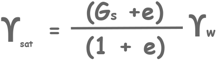substitute all these values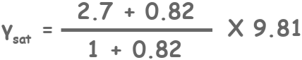γsat = 18.97 KN/m3

now substitute this into  equation number 3

18.97 x (6 - X) = 9.81 x 5

X = 3.4m

We can excavate the soil up to 3.4 meters under the ground.

Video version :

Tags : seepage

Published on :2020-08-23 04:45:21